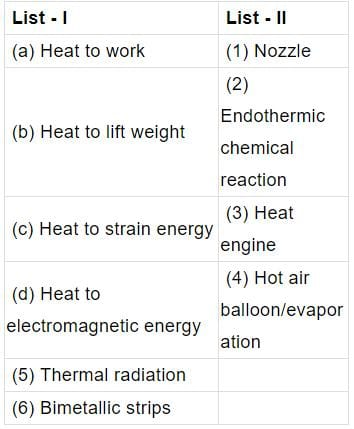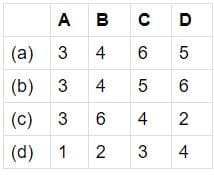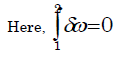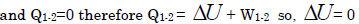Test: Basic Concepts of Thermodynamics - 1

# Test: Basic Concepts of Thermodynamics - 1

Test Description

## 20 Questions MCQ Test GATE Mechanical (ME) 2023 Mock Test Series | Test: Basic Concepts of Thermodynamics - 1

Test: Basic Concepts of Thermodynamics - 1 for Mechanical Engineering 2023 is part of GATE Mechanical (ME) 2023 Mock Test Series preparation. The Test: Basic Concepts of Thermodynamics - 1 questions and answers have been prepared according to the Mechanical Engineering exam syllabus.The Test: Basic Concepts of Thermodynamics - 1 MCQs are made for Mechanical Engineering 2023 Exam. Find important definitions, questions, notes, meanings, examples, exercises, MCQs and online tests for Test: Basic Concepts of Thermodynamics - 1 below.
Solutions of Test: Basic Concepts of Thermodynamics - 1 questions in English are available as part of our GATE Mechanical (ME) 2023 Mock Test Series for Mechanical Engineering & Test: Basic Concepts of Thermodynamics - 1 solutions in Hindi for GATE Mechanical (ME) 2023 Mock Test Series course. Download more important topics, notes, lectures and mock test series for Mechanical Engineering Exam by signing up for free. Attempt Test: Basic Concepts of Thermodynamics - 1 | 20 questions in 60 minutes | Mock test for Mechanical Engineering preparation | Free important questions MCQ to study GATE Mechanical (ME) 2023 Mock Test Series for Mechanical Engineering Exam | Download free PDF with solutions
 1 Crore+ students have signed up on EduRev. Have you?
Test: Basic Concepts of Thermodynamics - 1 - Question 1

###Codes:Detailed Solution for Test: Basic Concepts of Thermodynamics - 1 - Question 1

A heat engine is a system that converts heat into work by taking heat from the reservoir ( hot body) to carry out some work. There is a discharge of some heat to the sink (cold body).

Hot Air Balloon is used to lift the weight using heat.

bimetallic strip is used to convert a temperature change into mechanical displacement. The strip consists of two strips of different metals which expand at different rates as they are heated. ... This effect is used in a range of mechanical and electrical devices.

Test: Basic Concepts of Thermodynamics - 1 - Question 2

### Heat and work are

Detailed Solution for Test: Basic Concepts of Thermodynamics - 1 - Question 2

Heat and work depend upon the path traversed to move from one thermodynamic state to another. This is why they are known as path functions.

Test: Basic Concepts of Thermodynamics - 1 - Question 3

### A balloon containing an ideal gas is initially kept in an evacuated and insulated room. The balloon ruptures and the gas fills up the entire room. Which one of the following statements is TRUE at the end of above process?

Detailed Solution for Test: Basic Concepts of Thermodynamics - 1 - Question 3

It is free expansion. Since vacuum does not offer any resistance, there is no work transfer involved in free expansion.Test: Basic Concepts of Thermodynamics - 1 - Question 4

A system comprising a single phase is called a

Test: Basic Concepts of Thermodynamics - 1 - Question 5

The main cause of the irreversibility is

Detailed Solution for Test: Basic Concepts of Thermodynamics - 1 - Question 5

There are basically two reasons of irreversibility of a thermodynamic process.

1. Involvement of dissipative effect during the process.
2. Lack of thermodynamic equilibrium during the process.
Test: Basic Concepts of Thermodynamics - 1 - Question 6

Which of the following are intensive properties?

1. Kinetic Energy

2. Specific Enthalpy

3. Pressure

4. Entropy

Select the correct answer using the code given below:

Detailed Solution for Test: Basic Concepts of Thermodynamics - 1 - Question 6

Option (b) 2 and 3 is  correct.

Specific enthalpy and pressure

Are both intensive properties.

Explanation:-

{ Intensive properties are those that do not depend on the size of the system. Specific enthalpy is the enthalpy on a per gram basis. Since  this value is normalized to the per gram basis it does not depend on the size of the system being measured. }
So, both are intensive properties.

Test: Basic Concepts of Thermodynamics - 1 - Question 7

Consider the following:

1. Kinetic energy

2. Entropy

3. Thermal conductivity

4. Pressure

Which of these are intensive properties?

Detailed Solution for Test: Basic Concepts of Thermodynamics - 1 - Question 7

Kinetic energy 1/2mv2 depends on mass, Entropy kJ/k depends on mass so Entropy is extensive property but specific entropy kJ/kg K is an intensive property.

Test: Basic Concepts of Thermodynamics - 1 - Question 8

Consider the following properties:

1. Entropy

2. Viscosity

3. Temperature

4. Specific heat at constant volume

Which of the above properties of a system is/are extensive?

Detailed Solution for Test: Basic Concepts of Thermodynamics - 1 - Question 8

Extensive property: Whose value depends on the size or extent i.e. mass of the system (upper case letters as the symbols) e.g., Volume, Mass (V, M). If mass is increased, the value of extensive property also increases.

Test: Basic Concepts of Thermodynamics - 1 - Question 9

In highly rarefied gases, the concept of this loses validity

Test: Basic Concepts of Thermodynamics - 1 - Question 10

A control volume is

Detailed Solution for Test: Basic Concepts of Thermodynamics - 1 - Question 10

A control volume is a mathematical abstraction employed in the process of creating mathematical models of physical processes. In an inertial frame of reference, it is a volume fixed in space or moving with constant flow velocity through which the continuum (gas, liquid or solid) flows. The surface enclosing the control volume is referred to as the control surface.

At steady state, a control volume can be thought of as an arbitrary volume in which the mass of the continuum remains constant. As a continuum moves through the control volume, the mass entering the control volume is equal to the mass leaving the control volume. At steady state, and in the absence of work and heat transfer, the energy within the control volume remains constant. It is analogous to the classical mechanics concept of the free body diagram.

Test: Basic Concepts of Thermodynamics - 1 - Question 11

Isothermal compression of air in a Stirling engine is an example of

Test: Basic Concepts of Thermodynamics - 1 - Question 12

Assertion (A): In thermodynamic analysis, the concept of reversibility is that, a reversible process is the most efficient process.

Reason (R): The energy transfer as heat and work during the forward process as always identically equal to the energy transfer is heat and work during the reversal or the process.

Detailed Solution for Test: Basic Concepts of Thermodynamics - 1 - Question 12

The energy transfer as heat and work during the forward process as always
identically equal to the energy transfer is heat and work during the reversal or the process is the correct reason for maximum efficiency because it is conservative system.

Test: Basic Concepts of Thermodynamics - 1 - Question 13

Ice kept in a well insulated thermo flask is an example of which system?

Detailed Solution for Test: Basic Concepts of Thermodynamics - 1 - Question 13

Isolated System - in which there is no interaction between system and the
surroundings. It is of fixed mass and energy, and hence there is no mass and energy transfer across the system boundary.

Test: Basic Concepts of Thermodynamics - 1 - Question 14

A thermodynamic system is considered to be an isolated one if

Detailed Solution for Test: Basic Concepts of Thermodynamics - 1 - Question 14

A thermodynamic system is considered to be an isolated one if there is no energy and mass transfer taking place.

Test: Basic Concepts of Thermodynamics - 1 - Question 15

Measurement of temperature is based on which law of thermodynamics?

Detailed Solution for Test: Basic Concepts of Thermodynamics - 1 - Question 15

All temperature measurements are based on Zeroth law of thermodynamics.

Test: Basic Concepts of Thermodynamics - 1 - Question 16

Zeroth Law of thermodynamics states that

Detailed Solution for Test: Basic Concepts of Thermodynamics - 1 - Question 16

The Zeroth law of thermodynamics is the basis for measurement of temperature and setting its scale. In simple word, Zeroth law of thermodynamics says that “When two bodies are separately in thermal equilibrium with the third body, then the two are also in thermal equilibrium with each other."

Test: Basic Concepts of Thermodynamics - 1 - Question 17

The constant volume gas thermometer works on the principle that

Detailed Solution for Test: Basic Concepts of Thermodynamics - 1 - Question 17

We know that PV=mRT and it holds good at low pressure and high temperature. If volume is constant P is proportional to T (since R is constant and m depends on gas and is also constant). Therefore, at low pressure, the temperature of gas is proportional to its pressure at constant volume.

Test: Basic Concepts of Thermodynamics - 1 - Question 18

Assertion (a): If an alcohol and a mercury thermometer read exactly 0°C at the ice point and 100°C at the steam point and the distance between the two points is divided into 100 equal parts in both thermometers, the two thermometers will give exactly the same reading at 50°C.

Reason (R): Temperature scales are arbitrary.

Detailed Solution for Test: Basic Concepts of Thermodynamics - 1 - Question 18

Because firstly they are of different material they have different thermal expansion etc and secondly no 2 things whether of same company can tel u the same.
Eg. if u purchase the weighing machine of 2 companies even they can' t tell the same weight .. i.e some amt of deflection will be there

Test: Basic Concepts of Thermodynamics - 1 - Question 19

Pressure reaches a value of absolute zero

Detailed Solution for Test: Basic Concepts of Thermodynamics - 1 - Question 19

Absolute zero, temperature at which a thermodynamic system has the lowest energy. It appeared that an “ideal gas” at constant pressure would reach zero volume at what is now called the absolute zero of temperature.

Test: Basic Concepts of Thermodynamics - 1 - Question 20

Assertion (A): Thermodynamic work is path-dependent except for an adiabatic process.

Reason(R): It is always possible to take a system from a given initial state to any final state by performing adiabatic work only.

Detailed Solution for Test: Basic Concepts of Thermodynamics - 1 - Question 20

It is not possible to take a system from a given initial state to any final state by performing adiabatic work only.

The assertion is true since in an adiabatic process heat transfer is zero and work is equal to change in internal energy.

## GATE Mechanical (ME) 2023 Mock Test Series

27 docs|243 tests
 Use Code STAYHOME200 and get INR 200 additional OFF Use Coupon Code
Information about Test: Basic Concepts of Thermodynamics - 1 Page
In this test you can find the Exam questions for Test: Basic Concepts of Thermodynamics - 1 solved & explained in the simplest way possible. Besides giving Questions and answers for Test: Basic Concepts of Thermodynamics - 1, EduRev gives you an ample number of Online tests for practice

## GATE Mechanical (ME) 2023 Mock Test Series

27 docs|243 tests